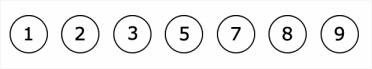GMAT Question of the Day - Daily to your Mailbox; hard ones only

 It is currently 20 Nov 2019, 09:53### GMAT Club Daily Prep

#### Thank you for using the timer - this advanced tool can estimate your performance and suggest more practice questions. We have subscribed you to Daily Prep Questions via email.

Customized
for You

we will pick new questions that match your level based on your Timer History

Track

every week, we’ll send you an estimated GMAT score based on your performance

Practice
Pays

we will pick new questions that match your level based on your Timer History

#### Not interested in getting valuable practice questions and articles delivered to your email? No problem, unsubscribe here.# Little Julia created a 5-digit integer choosing 5 distinct chips, one

Author Message
TAGS:

### Hide Tags

GMATH TeacherP
Status: GMATH founder
Joined: 12 Oct 2010
Posts: 935
Little Julia created a 5-digit integer choosing 5 distinct chips, one  [#permalink]

### Show Tags

1
300:00

Difficulty:55% (hard)

Question Stats:63% (02:22) correct38% (02:59) wrongbased on 32 sessions

### HideShow timer Statistics

GMATH practice exercise (Quant Class 18)Little Julia created a 5-digit integer choosing 5 distinct chips, one by one, among the 7 given ones shown above. "Can you do it in such a way that the three central digits add up to 9?", asked her teacher. And Julia did! If little Max was asked to do the same by his teacher, and Max chooses a correct possibility randomly, what is the probability that both children have chosen exactly the same 5-digit integer?

(A) 1/12
(B) 1/36
(C) 1/54
(D) 1/72
(E) 1/96

_________________
Fabio Skilnik :: GMATH method creator (Math for the GMAT)
Our high-level "quant" preparation starts here: https://gmath.net
ManagerG
Joined: 12 Apr 2011
Posts: 149
Location: United Arab Emirates
Concentration: Strategy, Marketing
Schools: CBS '21, Yale '21, INSEAD
GMAT 1: 670 Q50 V31GMAT 2: 720 Q50 V37WE: Marketing (Telecommunications)
Re: Little Julia created a 5-digit integer choosing 5 distinct chips, one  [#permalink]

### Show Tags

3
fskilnik wrote:
GMATH practice exercise (Quant Class 18)Little Julia created a 5-digit integer choosing 5 distinct chips, one by one, among the 7 given ones shown above. "Can you do it in such a way that the three central digits add up to 9?", asked her teacher. And Julia did! If little Max was asked to do the same by his teacher, and Max chooses a correct possibility randomly, what is the probability that both children have chosen exactly the same 5-digit integer?

(A) 1/12
(B) 1/36
(C) 1/54
(D) 1/72
(E) 1/96

Lets see the number of 5-digit integers that can be formed such that "three central digits add up to 9".

From the given digits only 5+3+1 = 9, hence the middle three digits are 5,3,1 and the for the remaining two digits we 4 options - 2, 7, 8, 9.

Hence for:

1st digit = 4 ways
5th digit = 3 ways
middle 3 digits can be formed in 3! ways as we have three digits
So total ways = 4*3*3*2*1 = 72 ways

Now if Julia selected a 6 digits number, Max can select the same number with a probability of 1/72 as only way will match out of 72.

Hence D is the correct answer.
_________________
Hit Kudos if you like what you see!
GMATH TeacherP
Status: GMATH founder
Joined: 12 Oct 2010
Posts: 935
Little Julia created a 5-digit integer choosing 5 distinct chips, one  [#permalink]

### Show Tags

fskilnik wrote:
GMATH practice exercise (Quant Class 18)Little Julia created a 5-digit integer choosing 5 distinct chips, one by one, among the 7 given ones shown above. "Can you do it in such a way that the three central digits add up to 9?", asked her teacher. And Julia did! If little Max was asked to do the same by his teacher, and Max chooses a correct possibility randomly, what is the probability that both children have chosen exactly the same 5-digit integer?

(A) 1/12
(B) 1/36
(C) 1/54
(D) 1/72
(E) 1/96

Very nice, eabhgoy! (Kudos!)

(Just a small correction: "Now if Julia selected a 5 digits number, Max can...")

Here is our official solution:

$$?\,\, = \,\,{1 \over {\# \,\,{\rm{favorable}}\,\,{\rm{sequences}}}}$$

$${?_{temp}}\,\,\, = \,\,\,\# \,\,{\rm{favorable}}\,\,{\rm{sequences}}$$

$$\left\{ \matrix{ \,{\rm{3}}\,{\rm{central}}\,{\rm{digits}}\,{\rm{are}}\,\,{\rm{1,3,5}}\,\,\,\, \Rightarrow \,\,\,{{\rm{P}}_{\rm{3}}} = 3!\,\,\,{\rm{possibilities}} \hfill \cr \,{\rm{first}}\,{\rm{and}}\,\,{\rm{last}}\,\,{\rm{digits}}\,\,\,{\rm{:}}\,\,\,{\rm{4}} \cdot {\rm{3}}\,\,{\rm{possibilities}} \hfill \cr} \right.\,\,\,\,\,\,\,\, \Rightarrow \,\,\,\,{?_{temp}}\,\,\, = \,\,\,3!\, \cdot 4 \cdot 3\,\, = \,\,\,72$$

We follow the notations and rationale taught in the GMATH method.

Regards,
Fabio.
_________________
Fabio Skilnik :: GMATH method creator (Math for the GMAT)
Our high-level "quant" preparation starts here: https://gmath.net
GMATH TeacherP
Status: GMATH founder
Joined: 12 Oct 2010
Posts: 935
Little Julia created a 5-digit integer choosing 5 distinct chips, one  [#permalink]

### Show Tags

eabhgoy wrote:
Thank you sir
Hi eabhgoy!

See you in other posts and success in your studies/activities!

Regards,
Fabio.
_________________
Fabio Skilnik :: GMATH method creator (Math for the GMAT)
Our high-level "quant" preparation starts here: https://gmath.netLittle Julia created a 5-digit integer choosing 5 distinct chips, one   [#permalink] 07 Mar 2019, 13:28
Display posts from previous: Sort by

# Little Julia created a 5-digit integer choosing 5 distinct chips, one